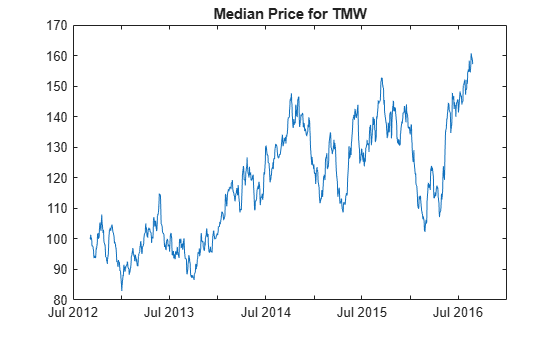# medprice

`medprice` has been partially removed and will no longer accept a `fints` object (`tsobj`) argument. Use a matrix, `timetable`, or `table` instead for financial time series.

Use `fts2timetable` to convert a `fints` object to a `timetable` object.

## Syntax

``MedianPrice = medprice(Data)``

## Description

example

````MedianPrice = medprice(Data)` calculates the median prices from the series of high and low prices. The median price is the average of the high and low prices for each period.```

## Examples

collapse all

Load the file `SimulatedStock.mat`, which provides a timetable (`TMW`) for financial data for TMW stock.

```load SimulatedStock.mat MedianPrice = medprice(TMW); plot(MedianPrice.Time,MedianPrice.MedianPrice) title('Median Price for TMW')```## Input Arguments

collapse all

Data for high, low, and close prices, specified as a matrix, table, or timetable. For matrix input, `Data` is an `M`-by-`3` matrix of high, low, and close prices stored in the corresponding columns. Timetables and tables with `M` rows must contain a variable named `'High'`, `'Low'`, `'Close'` (case insensitive).

Data Types: `double` | `table` | `timetable`

## Output Arguments

collapse all

Median price series, returned with the same number of rows (`M`) and the same type (matrix, table, or timetable) as the input `Data`.

 Achelis, S. B. Technical Analysis from A to Z. Second Edition. McGraw-Hill, 1995, pp. 177–178.